# GWB 環境地質分析軟體-資安軟體/研究分析軟體/心理學軟體/新永資訊有限公司

## GWB 環境地質分析軟體• GWB 環境地質分析軟體
• 類別
水文軟體
• 介紹
The Geochemist's Workbench®, is the integrated package which consists of the tool which the stability diagram of calculation and the natural water of the chemical reaction and calculation of state of equilibrium, trace, and these calculation results of reaction path to graph is converted
• 價格

### GWB  環境地質分析軟體

The Geochemist's Workbench®, is the integrated package which consists of the tool which the stability diagram of calculation and the natural water of the chemical reaction and calculation of state of equilibrium, trace, and these calculation results of reaction path to graph is converted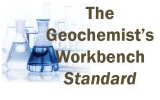## The main function of GWB:

•  • Calculation of reaction path
•  • Rate equation
•  • Division of isotope
•  • Adsorption and calculation of complex reaction of surface
•  • Brine chemistry, “Pitzer method”
•  • Temperature - activity diagram
•  • Calculation of chemical kind distribution diagram
•  • Immediately reaction equilibrium conversion, equilibrium constant an • d equation of equilibrium, calculation of temperature
•  • Compilation of Eh-pH/pe-pH/activity-activity diagram

The Geochemist's Workbench® consists of the program below.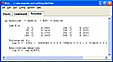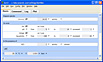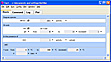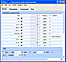Rxn Act2 Tact React Gtplot

•  • Rxn: Automatic equilibrium calculation, equilibrium constant of chemical reaction and calculation of formula and calculation of terminal temperature.
•  • Act2: Trace of the reaction path which was calculated by the calculation graph conversion and the reaction program of the stability diagram of activity.
•  • Tact: Temperature - activity and temperature - trace indication of calculation plotting and reaction path of volatilization diagram.
•  • SpecE8, saturated state of distribution and the mineral of chemical kind, fugacity of the vapor ([hugashitei]) does calculation, and the similar calculation regarding the natural water quickly.
•  • Aqplot draws the calculation result of SpecE8 as the graph. It can select graph type from midst of plural.
•  • React (Std/Pro): Trace of the reaction path which calculates the distribution of water 溶 ones, includes the liquid, the mineral and the vapor.
•  • Gtplot (Std/Pro): Also it is possible to indicate the reaction path which traces with React, to output the graph with the black and white or color printer, through the clipboard, the copy & the paste to do the diagram.
•  • X1t (Pro) - the module which executes 1D reaction transport simulation
•  • X2t (Pro) - the module which executes 2D reaction transport simulation
•  • Xtplot (Pro) - the tool which indicates the result of the simulation due to X1t and X2t
Comparison of module
 GWB GWB GWB Essentials Standard Professional Rxn ○ ○ ○ Act2 ○ ○ ○ Tact ○ ○ ○ SpecE8 ○ ○ ○ Aqplot ○ ○ ○ React ○ ○ Gtplot ○ ○ X1t ○ X2t ○ Xtplot ○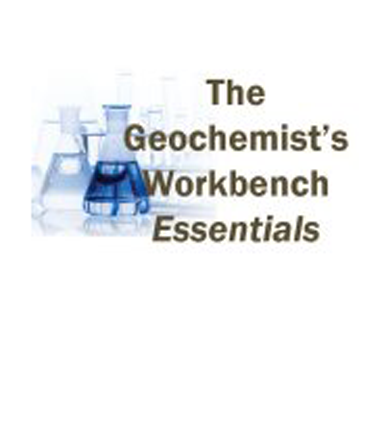### GMS 水文環境軟體### PORFLOW 地下水與核廢水管理軟體

PORFLOW - #1 choice of simulation software among consultants and researchers alike in the Ground Water Flow and Nuclear Waste Management fields - is here. On top of the many stability and versatility features built into the solver, PORFLOW comes bundled with CFDStudio™, the integrated development environment for CFD applications.### WMS 水文軟體

Watershed Modeling System(WMS)是非常有名的流域模擬分析軟體，在全程支持流域（分水嶺）的水文地理學，水力學的圖形化建模。是美國地質調查局和環保局批准的環境模擬軟體